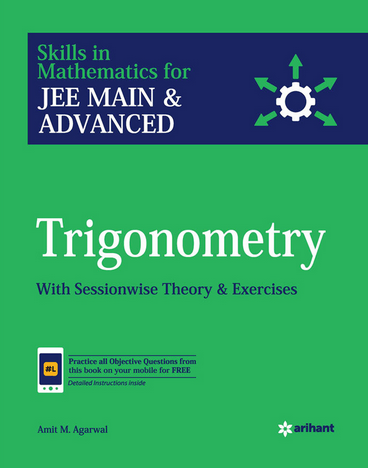# Trigonometry (Weightage 4%)   Share

### Topics from Trigonometry

• Trigonometrical functions, Inverse trigonometrical functions and their properties (30 concepts)
• Trigonometrical identities and equations (68 concepts)
• Height and distance (4 concepts)
• Trigonometric Ratios and Transformation Formulas (15 concepts)
• Trigonometric Functions (9 concepts)
• Trigonometric Equations (8 concepts)
• Properties and Solutions of Triangle (14 concepts)
• Inverse Trigonometric Functions (20 concepts)

### Important Books for Trigonometry

•••Exams
Articles
Questions